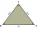# Parallelogram

Calculate the missing side of a parallelogram, if you know the perimeter o and one side:

a) o = 7.2 cm; b = 1.8 cm
b) o = 5.4 cm; a = 1.9 cm

Result

a =  1.8 cm
b =  0.8 cm

#### Solution:Leave us a comment of example and its solution (i.e. if it is still somewhat unclear...):

Showing 0 comments:Be the first to comment!## Next similar examples:

1. Arm-legCalculate the length of the base of an isosceles triangle with a circumference 224 cm if the arm length is 68 cm.
2. Perimeter to areaCalculate the area of a circle with the perimeter 15 meters.
3. AverageThe arithmetic mean of the two numbers is 71.7. One number is 5. Calculate the second number.
4. ClassIn 7.C clss are 10 girls and 20 boys. Yesterday was missing 20% of girls and 50% boys. What percentage of students missing?
5. Sides of triangleTriangle circumference with two identical sides is 117cm. The third side measures 44cm. How many cms do you measure one of the same sides?
6. CircleWhat is the radius of the circle whose perimeter is 6 cm?
7. MineWheel in traction tower has a diameter 5 m. How many meters will perform an elevator cabin if wheel rotates in the same direction 49 times?
8. HotelThe hotel has a p floors each floor has i rooms from which the third are single and the others are double. Represents the number of beds in hotel.
9. AlleyAlley measured a meters. At the beginning and end are planted poplar. How many we must plant poplars to get the distance between the poplars 15 meters?
10. Simplify 2Simplify expression: 5ab-7+3ba-9
11. GivenGiven 2x =0.125 find the value of x
12. SimplifySimplify the following problem and express as a decimal: 5.68-[5-(2.69+5.65-3.89) /0.5]
13. Greg and BillGreg is 18 years old. He is 6 less than 4 times Bill's age. How old is Bill?
14. Boys and girlsThere are 28 girls in the hall. 5/7 of all children are boys. How many children and how many boys are there?
15. Obtuse angleWhich obtuse angle is creating clocks at 17:00?
16. IronIron ore contains 57% iron. How much ore is needed to produce 20 tons of iron?
17. Seeds 2How many seeds germinated from 1000 pcs, when 23% no emergence?